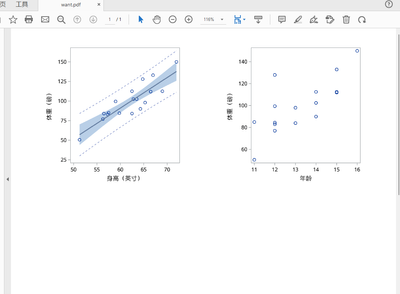## How to use ODS LAYOUT GRIDDED with ODS DOCUMENT

Let's say I create two plots and send them to ODS DOCUMENT. Then I want the output to appear as two side by side plots, I am trying to accomplish this via ODS LAYOUT GRIDDED and PROC DOCUMENT. But the two plots never show up side by side, they always show us one plot above the other plots. I have tried to do this with the output of PROC DOCUMENT going to HTML, doesn't work; I have tried to do this with the output of PROC DOCUMENT going to PDF, doesn't work.

How do I get this to show up as two plots side by side? Here is the PDF code. The HTML code is an obvious modification of the code below.

``````ods graphics/height=3in width=4in;
ods document name=work.test(write);
proc sgplot data=sashelp.class;
scatter x=height y=weight;
run;
proc sgplot data=sashelp.class;
scatter x=age y=weight;
run;
ods document close;

proc document name=work.test;
list/levels=all;
run;
quit;

ods pdf file="d:\myfolder\temp\plots.pdf" ;
ods layout gridded columns=2 rows=1 advance=proc;
replay \sgplot#1\sgplot#1 / dest=pdf;
run;
replay \sgplot#2\sgplot#1 / dest=pdf;
run;
ods layout end;
ods pdf close;``````

Thanks!

--
Paige Miller
1 ACCEPTED SOLUTION

Accepted Solutions

## Re: How to use ODS LAYOUT GRIDDED with ODS DOCUMENT

Try adding ODS REGION statements between each region you want in the layout.

``````ods layout gridded columns=2 rows=1 advance=proc;
ods region;
replay \sgplot#1\sgplot#1 / dest=pdf;
run;
ods region;
replay \sgplot#2\sgplot#1 / dest=pdf;
run;
ods layout end;``````
5 REPLIES 5

## Re: How to use ODS LAYOUT GRIDDED with ODS DOCUMENT

Try adding ODS REGION statements between each region you want in the layout.

``````ods layout gridded columns=2 rows=1 advance=proc;
ods region;
replay \sgplot#1\sgplot#1 / dest=pdf;
run;
ods region;
replay \sgplot#2\sgplot#1 / dest=pdf;
run;
ods layout end;``````DanH_sas
SAS Super FREQ

## Re: How to use ODS LAYOUT GRIDDED with ODS DOCUMENT

@ChrisHemedinger is correct. You can also drop the ADVANCE=PROC option in your example. I'm not sure why that option is not working for PROC DOCUMENT, but using ODS REGION statements should always create a clear cell delineations.

## Re: How to use ODS LAYOUT GRIDDED with ODS DOCUMENT

Well, that was simple. Thank you!

--
Paige MillerKsharp
Super User

## Re: How to use ODS LAYOUT GRIDDED with ODS DOCUMENT

``````/*Here is a alternative way.*/
%let path= c:\temp ;

options nodate nonumber;
ods listing gpath="&path."  style=htmlblue image_dpi=300;
ods graphics /width=3in height=3in reset=index  noborder  imagename='FAS' outputfmt=png ;
proc sgplot data=sashelp.class noautolegend ;
reg x=height y=weight/cli clm;
run;

ods graphics /width=3in height=3in reset=index  noborder  imagename='PPS' outputfmt=png;
proc sgplot data=sashelp.class;
scatter x=age y=weight;
run;

ods pdf file="&path.\want.pdf" style=minimal dpi=300  ;
data x;
x=' ';
y=' ';
run;
title;
proc report data=x  nowd noheader style={outputwidth=100% };
column x y;
define x/ style={ preimage="&path\FAS1.png"  bordertopcolor=white borderbottomcolor=white borderrightcolor=white borderleftcolor=white};
define y/ style={ preimage="&path\PPS1.png"  bordertopcolor=white borderbottomcolor=white borderrightcolor=white borderleftcolor=white};
run;

ods pdf close;``````Ksharp
Super User

## Re: How to use ODS LAYOUT GRIDDED with ODS DOCUMENT

``````%let path= c:\temp ;

options nodate nonumber;
ods listing gpath="&path."  style=htmlblue image_dpi=300;
ods graphics /width=3in height=3in reset=index  noborder  imagename='FAS' outputfmt=png ;
proc sgplot data=sashelp.class noautolegend ;
reg x=height y=weight/cli clm;
run;

ods graphics /width=3in height=3in reset=index  noborder  imagename='PPS' outputfmt=png;
proc sgplot data=sashelp.class;
scatter x=age y=weight;
run;

ods graphics /width=3in height=3in reset=index  noborder  imagename='SS' outputfmt=png ;
proc sgplot data=sashelp.class noautolegend ;
ellipse x=height y=weight/group=sex;
scatter x=height y=weight/group=sex;
run;

ods graphics /width=3in height=3in reset=index  noborder  imagename='S' outputfmt=png;
proc sgplot data=sashelp.class;
scatter x=age y=weight/group=sex datalabel=name;
run;

ods pdf file="&path.\want.pdf" style=minimal dpi=300  ;
data x;
x=' ';y=' ';output;
x=' ';y=' ';output;
run;
title;
proc report data=x  nowd noheader style={outputwidth=100% };
column x y;
define x/display;
define y/display;
compute y;
n+1;
if n=1 then do;
call define('x','style','style={ preimage="&path\FAS1.png"  bordertopcolor=white borderbottomcolor=white borderrightcolor=white borderleftcolor=white}');
call define('y','style','style={ preimage="&path\PPS1.png"  bordertopcolor=white borderbottomcolor=white borderrightcolor=white borderleftcolor=white}');
end;
if n=2 then do;
call define('x','style','style={ preimage="&path\SS1.png"  bordertopcolor=white borderbottomcolor=white borderrightcolor=white borderleftcolor=white}');
call define('y','style','style={ preimage="&path\S1.png"  bordertopcolor=white borderbottomcolor=white borderrightcolor=white borderleftcolor=white}');
end;
endcomp;
run;

ods pdf close;``````Discussion stats
• 5 replies
• 358 views
• 3 likes
• 4 in conversation